# HRRN Scheduling | CPU Scheduling

## HRRN Scheduling-

In HRRN Scheduling,

• Out of all the available processes, CPU is assigned to the process having highest response ratio.
• In case of a tie, it is broken by FCFS Scheduling.
• It operates only in non-preemptive mode.

## Calculating Response Ratio-

Response Ratio (RR) for any process is calculated by using the formula-where-

• W = Waiting time of the process so far
• B = Burst time or Service time of the process

• It performs better than SJF Scheduling.
• It not only favors the shorter jobs but also limits the waiting time of longer jobs.

• It can not be implemented practically.
• This is because burst time of the processes can not be known in advance.

## Problem-

Consider the set of 5 processes whose arrival time and burst time are given below-

 Process Id Arrival time Burst time P0 0 3 P1 2 6 P2 4 4 P3 6 5 P4 8 2

If the CPU scheduling policy is Highest Response Ratio Next, calculate the average waiting time and average turn around time.

## Solution-

### Step-01:

• At t = 0, only the process P0 is available in the ready queue.
• So, process P0 executes till its completion.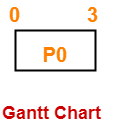### Step-02:

• At t = 3, only the process P1 is available in the ready queue.
• So, process P1 executes till its completion.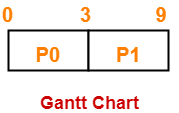### Step-03:

• At t = 9, the processes P2, P3 and P4 are available in the ready queue.
• The process having the highest response ratio will be executed next.

The response ratio are-

• Response Ratio for process P2 = [(9-4) + 4] / 4 = 9 / 4 = 2.25
• Response Ratio for process P3 = [(9-6) + 5] / 5 = 8 / 5 = 1.6
• Response Ratio for process P4 = [(9-8) + 2] / 2 = 3 / 2 = 1.5

Since process P2 has the highest response ratio, so process P2 executes till completion.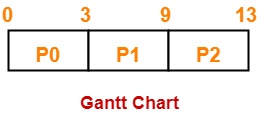### Step-04:

• At t = 13, the processes P3 and P4 are available in the ready queue.
• The process having the highest response ratio will be executed next.

The response ratio are calculated as-

• Response Ratio for process P3 = [(13-6) + 5] / 5 = 12 / 5 = 2.4
• Response Ratio for process P4 = [(13-8) + 2] / 2 = 7 / 2 = 3.5

Since process P4 has the highest response ratio, so process P4 executes till completion.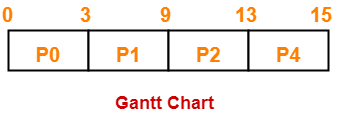### Step-05:

• At t = 15, only the process P3 is available in the ready queue.
• So, process P3 executes till its completion.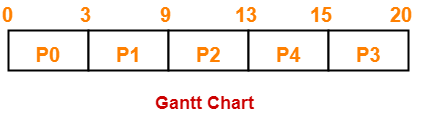Now, we know-

• Turn Around time = Exit time – Arrival time
• Waiting time = Turn Around time – Burst time

Also read- Various Times of Process

 Process Id Exit time Turn Around time Waiting time P0 3 3 – 0 = 3 3 – 3 = 0 P1 9 9 – 2 = 7 7 – 6 = 1 P2 13 13 – 4 = 9 9 – 4 = 5 P3 20 20 – 6 = 14 14 – 5 = 9 P4 15 15 – 8 = 7 7 – 2 = 5

Now,

• Average Turn Around time = (3 + 7 + 9 + 14 + 7) / 5 = 40 / 5 = 8 units
• Average waiting time = (0 + 1 + 5 + 9 + 5) / 5 = 20 / 5 = 4 units

To gain better understanding about HRRN Scheduling,

Watch this Video Lecture

Next Article- Round Robin Scheduling

Get more notes and other study material of Operating System.

Watch video lectures by visiting our YouTube channel LearnVidFun.

SummaryArticle Name
HRRN Scheduling | CPU Scheduling
Description
HRRN Scheduling or Highest Response Ratio Next Scheduling is a CPU scheduling algorithm that assigns CPU to the process having highest response ratio. Highest Response Ratio Next Example.
Author
Publisher Name
Gate Vidyalay
Publisher Logo